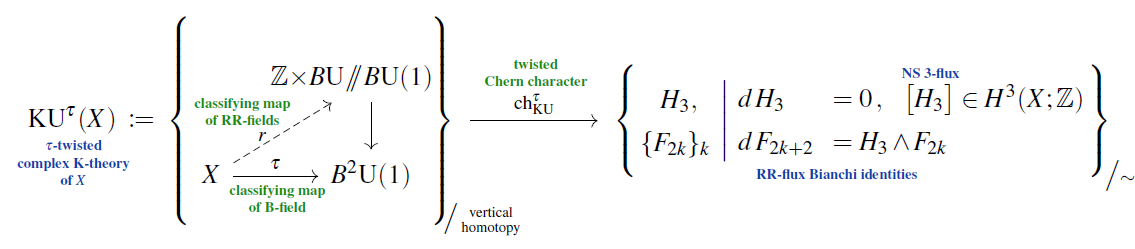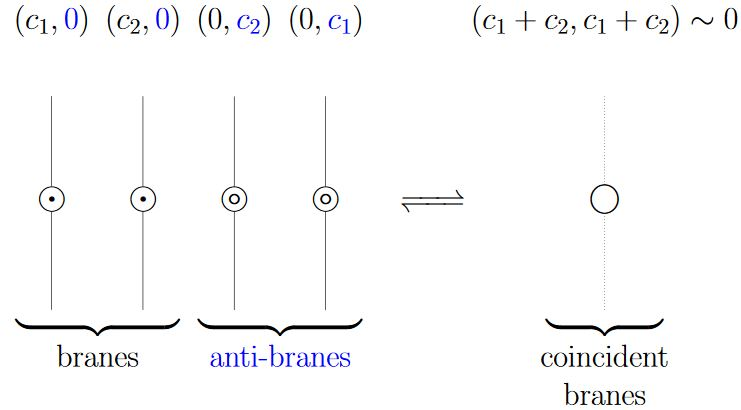Contrents

cohomology

# Contrents

## Idea

### Statement

A famous conjecture in non-perturbative string theory says (Minasian-Moore 97, Witten 98, reviewed in Witten 00, Moore 02, Manjarín 04, Evslin 06, Fredenhagen 08) that D-brane charge and RR-field fluxes are quantized in the Whitehead-generalized cohomology theory known as topological K-theory, specifically in KU-cohomology for type II string theory and more generally in KR cohomology theory in the presence of orientifolds, reducing to KO-cohomology in type I string theory right on the O-planes.

More generally, the conjecture says that the fluxes of the NS B-field and of the RR-fields are jointly flux-quantized in twisted topological K-theory, with the B-field constituting the twist.

Yet more generally, the conjecture says that, over orbifolds, the charge quantization is in orbifold K-theory, hence in equivariant K-theory for the usual global quotient orbifolds, hence in twisted equivariant K-theory for the combined B-field/RR-fields. A sketch of how to state this more precisely is in Distler-Freed-Moore 09.

This means, or would mean, that the full massless physical fields in type II string theory, including their higher “gauge potentials”, are cocycles in a differential cohomology-version of these twisted generalized cohomology theories, hence in differential K-theory if the B-field is ignored, in twisted differential K-theory if the B-field is included, and in twisted equivariant differential K-theory if also orbifolds are taken into account.

### Motivation

An immediate way to motivate the conjecture is to notice that the image of the $H^3$-twisted Chern character on twisted K-theory (say in degree 0, hence in type IIA string theory, for definitess)(from SS20)

takes values in $H_3$-twisted de Rham cohomology, whose cocycles are collections of differential forms satisfying exactly the Bianchi identities

$d \, H_3 = 0 \,, \;\;\; d\, F_{2k + 2} = H_3 \wedge F_{2k}$

expected of the B-field flux $H_3$ and the RR-field fluxes $F_{2k}$. This is ultimately what underlies the observation in Minasian-Moore 97, as brought out clearly in Brodzki-Mathai-Rosenberg-Szabo 06.From Sch 18

A conceptual picture of why topological K-theory would capture D-brane charge was suggested in Witten 98, Section 3: The tachyon condensation expected (this is Sen's conjecture from Sen 98) for open strings between D-brane/anti-D-branes plausibly implements on their Chan-Paton vector bundles the defining equivalence relation (here) of topological K-theory, as indicated in the figure.

While plausible and widely accepted in the string theory folklore, this picture remains to be checked (see below).

## Open problems

While the conjecture that D-brane charge is in K-theory has become widely accepted folklore in much of the string theory-community, it remains unproven – just as many and most folklore-statements involving non-perturbative brane-physics, not the least because an actual theory of non-perturbative string theory (aka M-theory) remains missing (see also at M-theory – the open problem).

Moreover, there are a number of indications that the conjecture can not actually be true without some modification, as in parts it seems to be in contradiction with other accepted facts about string theory. We list some of these issues in the following.

Since the full non-perturbative M-theory-completion of string theory is thought to involve not D-branes coupled to the B-field/RR-fields], but their lift (through the duality between type IIA string theory and M-theory) to M-branes coupled to the C-field, what is arguably missing (BDHKMMS 01, Sec. 4.6.5) is an M-brane/C-field charge/flux quantization law, which would reduce to something close to K-theoretic charge quantization in the appropriate limit. See the last item below.

### Checking Sen’s conjecture

While the proposal of Witten 98, Section 3 to explain the K-theory charge quantization conjecture via Sen's conjecture on tachyon condensation for open superstrings stretched between D-brane/anti D-brane pairs has become widely accepted folklore, the closest available towards an actual check of this argument via open superstring tachyon condensation seems to be Erler 13 which, however, concludes (on p. 32) with:

It would also be interesting to see if these developments can shed light on the long-speculated relation between string field theory and the K-theoretic description of D-brane charge $[$75, 76, 77$]$. We leave these questions for future work.

See also Erler 19 which still lists this (on p. 112) among open problems of string field theory:

Are there topological invariants of the open string star algebra representing D-brane charges?

### Incompatibility with S-duality

Taken at face value, the K-theory conjecture applied to type IIB string theory breaks the expected S-duality symmetry of the theory: Under this duality the B-field $H_3$-flux form and the RR-field 3-flux $F_3$ appear on the same footing and in particular mix as linear combinations (jointly coupling to (p,q)-strings), while in twisted K-theory $H_3$ is part of the twist, while $F_3$ is part of what is being twisted, with no evident way to mix these two concepts.

(see also Evslin 06, Sec. 8.3)

### Spurious fractional D-brane charges

An old argument of BDHKMMS 01, Sec. 4.5.2 says that equivariant K-theory by itself produces spurious fractional D-brane charges that need to be eliminated by some modification of the K-theory conjecture.

Evidence that this correction is provided by lifting to equivariant stable Cohomotopy instead (Hypothesis H) is offered in BSS 20.

### Spurious D-brane charges on group manifolds

Similarly, the analysis of boundary conformal field theory describing open strings on Lie groups (WZW models) does not seem to always agree with the conjectured K-theory classification of the corresponding D-branes:

It might surprise that despite all the progress that has been made in understanding branes on group manifolds, there are usually not enough D-branes known to explain the whole charge group predicted by (twisted) K-theory.

### Lift to M-theory

Finally, the lift of the putative D-brane charge quantization in string theory to an M-brane charge quantization in M-theory has remained largely open and problematic, as maybe first highlighted in BDHKMMS 01, Sec. 4.6.5 for a variety of reasons.

However, a highly non-trivial check in DMW 00 successfully matched the first non-trivial differential in the Atiyah-Hirzebruch spectral sequence for topological K-theory, applied on the RR-flux component $F_4$, to a constraint on the C-fied flux $G_4$ obtained from a path integral-argument in D=11 supergravity.

Incidentally, this and related conditions on the C-field derived in DMW 00 from a path integral-argument in D=11 supergravity are all implied also by the Hypothesis H that the C-field, in turn, is charge quantized in twisted Cohomotopy theory (FSS 19).

## Properties

### D-brane charge quantization in topological K-theory

On the conjectural D-brane charge quantization in topological K-theory:

#### Origin and basics

The idea that D-branes have Dirac charge quantization in topological K-theory originates with:

Here:From Sch 18

Expression of these D-brane K-theory classes via the Atiyah-Hirzebruch spectral sequence:

Specifically for D-branes in WZW models see

• Peter Bouwknegt, A note on equality of algebraic and geometric D-brane charges in WZW models (pdf)

Discussion of D-brane matrix models taking these K-theoretic effects into account (K-matrix model) is in

• T. Asakawa, S. Sugimoto, S. Terashima, D-branes, Matrix Theory and K-homology, JHEP 0203 (2002) 034 (arXiv:hep-th/0108085)

### Twisted, equivariant and differential refinement

Discussion of charge quantization in twisted K-theory for the case of non-vanishing B-field:

An elaborate proposal for the correct flavour of equivariant KR-theory needed for orientifolds is sketched in:

Discussion of full-blown twisted differential K-theory and its relation to D-brane charge in type II string theory

Discussion of full-blown twisted differential orthogonal K-theory and its relation to D-brane charge in type I string theory (on orientifolds):

#### Reviews

See also for instance

Discussion of D-branes in KK-theory is reviewed in

based on

In particular (BMRS2) discusses the definition and construction of D-brane charge as a generalized index in KK-theory. The discussion there focuses on the untwisted case. Comments on the generalization of this to topologicall non-trivial B-field and hence twisted K-theory is in

#### Conceptual problems

But there remain conceptual issues with the proposal that D-brane charge is in K-theory, as highlighted in

In particular, actual checks of the proposal that D-brane charge is given by K-theory, via concrete computation in boundary conformal field theory, have revealed some subtleties:

• Stefan Fredenhagen, Thomas Quella, Generalised permutation branes, JHEP 0511:004, 2005 (arXiv:hep-th/0509153)

It might surprise that despite all the progress that has been made in understanding branes on group manifolds, there are usually not enough D-branes known to explain the whole charge group predicted by (twisted) K-theory.

The closest available towards an actual check of the argument for K-theory via open superstring tachyon condensation (Witten 98, Section 3) seems to be

• Theodore Erler, Analytic Solution for Tachyon Condensation in Berkovits’ Open Superstring Field Theory, JHEP 1311 (2013) 007 (arXiv:1308.4400)

which, however, concludes (on p. 32) with:

It would also be interesting to see if these developments can shed light on the long-speculated relation between string field theory and the K-theoretic description of D-brane charge $[$75, 76, 77$]$. We leave these questions for future work.

See also

which still lists (on p. 112) among open problems of string field theory:

“Are there topological invariants of the open string star algebra representing D-brane charges?”

#### For orbifolds in equivariant K-theory

The proposal that D-brane charge on orbifolds is measured in equivariant K-theory (orbifold K-theory) goes back to

It was pointed out that only a subgroup of equivariant K-theory can be physically relevant in

Further discussion of equivariant K-theory for D-branes on orbifolds includes the following:

Discussion of real K-theory for D-branes on orientifolds includes the following:

The original observation that D-brane charge for orientifolds should be in KR-theory is due to

and was then re-amplified in

With further developments in

Discussion of orbi-orienti-folds using equivariant KO-theory is in

Discussion of the alleged K-theory classification of D-brane charge in relation to the M-theory C-field is in

See also

More complete discussion of double dimensional reduction of the supergravity C-field in 11d to the expected B-field and RR-field flux forms in 10d: